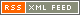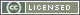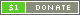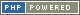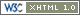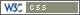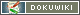# round

Rounds a Number.

Syntax

• `Number round( Number subject [, Number places [, Number method ] ] )`

Parameters

• Number `subject` - The number to round.
• Number `places` - The number of places to round to. If positive, that many decimal places are used to round to (if applicable). If negative, the rounding is done the opposite way.
• Number `method` - The rounding method to use.
• `0` (default) - Standard rounding will be used (nearest number).
• `1` - All numbers will be rounded down.
• `2` - All numbers will be rounded up.

Result

• Number - The rounded number.

Returns `subject` rounded to a specific number of places.